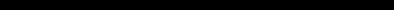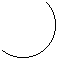# SINCLAIR ZX SPECTRUM BASIC ProgrammingCHAPTER 17
Graphics

#### Summary

PLOT, DRAW, CIRCLE, POINT, pixels

In this chapter we shall see how to draw pictures on the ZX Spectrum. The part of the screen you can use has 22 lines and 32 columns, making 22*32=704 character positions. As you may remember from Chapter 16, each of these character positions is made of an 8 by 8 square of dots, and these are called pixels (picture elements).

A pixel is specified by two numbers, its coordinates. The first, its x coordinate, says how far it is across from the extreme left-hand column. (Remember, x is a cross), the second, its y coordinate, says how far it is up from the bottom (wise up). These coordinates are usually written as a pair in brackets, so (0,0), (255,0), (0,175) and (255,175) are the bottom left-, bottom right-, top left- and top right-corners.

The statement

PLOT x coordinate, y coordinate

inks in the pixel with these coordinates, so this measles program

10 PLOT INT (RND*256), INT(RND*176): INPUT a\$: GO TO 10

plots a random point each time you press ENTER.

Here is a rather more interesting program. It plots a graph of the function SIN (a sine wave) for values between 0 and 2pi.

10 FOR n=0 TO 255
20 PLOT n,88+80*SlN (n/128*PI)
30 NEXT n

This next program plots a graph of SQR (part of a parabola) between 0 and 4:

10 FOR n=0 TO 255
20 PLOT n,80*SQR (n/64)
30 NEXT n

Notice that pixel coordinates are rather different from the line and column in an AT item. You may find the diagram in Chapter 15 useful when working out pixel coordinates and line and column numbers.

To help you with your pictures, the computer will draw straight lines, circles and parts of circles for you, using the DRAW and CIRCLE statements.

The statement DRAW to draw a straight line takes the form

DRAW x,y

The starting place of the line is the pixel where the last PLOT, DRAW or CIRCLE statement left off (this is called the PLOT position; RUN, CLEAR, CLS and NEW reset it to the bottom left hand corner, at (0,0)), and the finishing place is x pixels to the right of that and y pixels up. The DRAW statement on its own determines the length and direction of the line, but not its starting point.

Experiment with a few PLOT and DRAW commands, for instance

PLOT 0,100: DRAW 80,-35
PLOT 90,150: DRAW 80,-35

Notice that the numbers in a DRAW statement can be negative, although those in a PLOT statement can't.

You can also plot and draw in colour, although you have to bear in mind that colours always cover the whole of a character position and cannot be specified for individual pixels. When a pixel is plotted, it is set to show the full ink colour, and the whole of the character position containing it is given the current ink colour. This program demonstrates this:

10 BORDER 0: PAPER 0: INK 7: CLS: REM black out screen
20 LET x1=0: LET y1=0: REM start of line
30 LET c=1: REM for ink colour, starting blue
40 LET x2=lNT (RND*256): LET y2=lNT (RND*176): REM random finish of line
50 DRAW INK c;x2-x1,y2-y1
60 LET x1=x2: LET y1=y2: REM next line starts where last one finished
70 LET c=c+1: IF c=8 THEN LET c=1: REM new colour
80 GO TO 40

The lines seem to get broader as the program goes on, and this is because a line changes the colours of all the inked in pixels of all the character positions that it passes through. Note that you can embed PAPER, INK, FLASH, BRIGHT, INVERSE and OVER items in a PLOT or DRAW statement just as you could with PRINT and INPUT. They go between the key word and the coordinates, and are terminated by either semicolons or commas.

An extra frill with DRAW is that you can use it to draw parts of circles instead of straight lines, by using an extra number to specify an angle to be turned through: the form is

DRAW x,y,a

x and y are used to specify the finishing point of the line just as before and a is the number of radians that it must turn through as it goes - if a is a positive it turns to the left, while if a is a negative it turns to the right. Another way of seeing a is as showing the fraction of a complete circle that will be drawn: a complete circle is 2n radians, so if a= pi it will draw a semicircle, if a=0.5*pi a quarter of a circle, and so on.

For instance suppose a=pi. Then whatever values x and y take, a semicircle will be drawn. Run

10 PLOT 100,100: DRAW 50,50, Pl

which will draw this:
 finish at (150,150)start at (100,100)
The drawing starts off in a south-easterly direction, but by the time it stops it is going north-west: in between it has turned round through 180 degrees, or pi radians (the value of a).

Run the program several times, with PI replaced by various other expressions e.g. -PI, PI/2, 3*PI/2, PI/4, 1,0.

The last statement in this chapter is the CIRCLE statement, which draws an entire circle. You specify the coordinates of the centre and the radius of the circle using CIRCLE x coordinate, y coordinate, radius

Just as with PLOT and DRAW, you can put the various sorts of colour items in at the beginning of a CIRCLE statement.

The POINT function tells you whether a pixel is ink or paper colour. It has two arguments, the coordinates of the pixel (and they must be enclosed in brackets); and its result is 0 if the pixel is paper colour, 1 if it is ink colour. Try

CLS: PRINT POINT (0,0): PLOT 0,0: PRINT POINT (0,0)

Type

PAPER 7: INK 0

and let us investigate how INVERSE and OVER work inside a PLOT statement. These two affect just the relevant pixel, and not the rest of the character positions. They are normally off (0) in a PLOT statement, so you only need to mention them to turn them on (1).

Here is a list of the possibilities for reference:

PLOT; - this is the usual form. It plots an ink dot, i.e. sets the pixel to show the ink colour.

PLOT INVERSE 1; - this plots a dot of ink eradicator, i.e. it sets the pixel to show the paper colour.

PLOT OVER 1; - this changes the pixel over from whatever it was before: so if it was ink colour it becomes paper colour, and vice versa.

PLOT INVERSE 1; OVER 1; - this leaves the pixel exactly as it was before; but note that it also changes the PLOT position, so you might use it simply to do that.

As another example of using the OVER statement fill the screen up with writing using black on white, and then type
PLOT 0,0: DRAW OVER 1;255,175

This will draw a fairly decent line, even though it has gaps in it wherever it hits some writing. Now do exactly the same command again. The line will vanish without leaving any traces whatsoever. This is the great advantage of OVER 1. If you had drawn the line using PLOT 0,0: DRAW 255,175

and erased it using

PLOT 0,0: DRAW INVERSE 1;255,175

then you would also have erased some of the writing.

Now try

PLOT 0,0: DRAW OVER 1;250,175

and try to undraw it by

DRAW OVER 1;-250,-175

This doesn't quite work, because the pixels the line uses on the way back are not quite the same as the ones that it used on the way down. You must undraw a line in exactly the same direction as you drew it.

One way to get unusual colours is to speckle two normal ones together in a single square, using a user-defined graphic. Run this program:

1000 FOR n=0 TO 6 STEP 2
1010 POKE USR "a"+n, BIN 01010101: POKE USR "a"+n+1, BIN 10101010
1020 NEXT n

which gives the user-defined graphic corresponding to a chessboard pattern. If you print this character (graphics mode, then a) in red ink on yellow paper, you will find it gives a reasonably acceptable orange.

#### Exercises

 1. Play about with PAPER, INK, FLASH and BRIGHT items in a PLOT statement. These are the parts that affect the whole of the character position containing the pixel. Normally it is as though the PLOT statement had started off PLOT PAPER 8; FLASH 8; BRIGHT 8; ... and only the ink colour of a character position is altered when something is plotted there, but you can change this if you want. Be especially careful when using colours with INVERSE 1. because this sets the pixel to show the paper colour, but changes the ink colour and this might not be what you expect. 2. Try to draw circles using SIN and COS (if you have read Chapter 10, try to work out how). Run this: 10 FOR n=0 TO 2*PI STEP Pl /180 20 PLOT 100+80*COS n,87+80*SlN n 30 NEXT n 40 CIRCLE 150,87,80 You can see that the CIRCLE statement is much quicker, even if less accurate. 3. Try CIRCLE 100,87,80: DRAW 50,50 You can see from this that the CIRCLE statement leaves the PLOT position at a rather indeterminate place - it is always somewhere about half way up the right hand side of the circle. You will usually need to follow the CIRCLE statement with a PLOT statement before you do any more drawing. 4. Here is a program to draw the graph of almost any function. It first asks you for a number n; it will plot the values from -n to +n. It then asks you for the function itself, input as a string. The string should be an expression using x as the argument of the function. 10 PLOT 0,87: DRAW 255,0 20 PLOT 127,0: DRAW 0,175 30 INPUT s, e\$ 35 LET t=0 40 FOR f=0 TO 255 50 LET x=(f-128)*s/128: LET y=VAL e\$ 60 IF ABS y>87 THEN LET t=0: GO TO 100 70 IF NOT t THEN PLOT f,y+88: LET t=1: GO TO 100 80 DRAW 1,y-old y 100 LET old y=INT (y+.5) 110 NEXT f Run it and, as an example, type in 10 for the number n and 10*TAN x for the function. It will plot a graph of tan x as x ranges from -10 to +10.